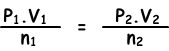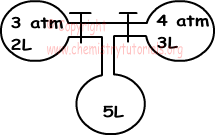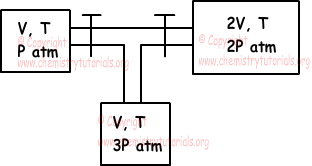## Gases Exam3 and Problem Solutions

Gases Exam3 and  Problem Solutions

1. Find volume of 0,5mol CH4 under 3,28 atm pressure and 400 0K temperature.

Solution:

P=3,28 atm, n=0,5mol, T=400 0K, R=0,082, V=?

We use ideal gas law;

P.V=n.R.T

3,28.V=0,5.0,082.400

V=5 liters

2. If 6,4 g CH4 has pressure 0,5 atm and volume 2 liters, find pressure of 9 g C2H6 having 1 liter volume under constant temperature.(C=12, H=1)

Solution:

We first find mole of given matters;

nCH4=6,4/16=0,4mol

nC2H6=9/30=0,3mol

Since temperature is constant we write ideal gas law as given below;(0,5.2)/0,4=(P2.1)/0,3

P2=0,75 atm

or P2= 57 cm Hg

3. Find density of O2 under 27 0C temperature and 1,23 atm pressure. (O=16)

Solution:

T=27 + 273=300 0K

If we write ideal gas law for density, we get following equation;

d=(P.M)/(R.T)

where M is molar mass of O2.

d=(1,23.32)/(0,082.300)

d=1,6g/liter

4. If we open the taps given in the picture below, find final temperature of gases.Solution:

We use following equation to find final pressure of gas mixture;

P1.V1 + P2.V2 + P3.V3 = Pfinal.Vfinal

3.2 + 4.3 + 0.5 =Pfinal.(2+3+5)

6 + 12=Pfinal.10

Pfinal=1,8 atm

5. When we open the taps given in the picture below, find changes in the pressures of gases.Solution:

We should find final pressure of system to make comparison.

P1.V1 + P2.V2 + P3.V3 = Pfinal.Vfinal

P.V + 2P.2V + 3P.V = Pfinal.(V+2V+V)

Pfinal=2P

Thus,

I. Pressure of first container increase

II. Pressure of second container stays constant

III. Pressure of third container decreases

Related